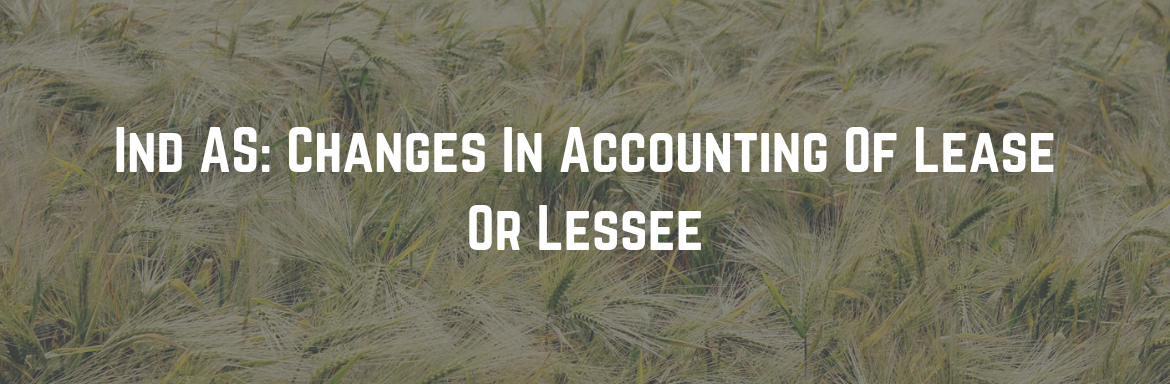# About Ind AS 116, Leases

The lessor and lessee will apply Ind AS 116 for accounting in their books. The principles of Ind AS 116 for lessor are same as Standard (Ind AS 17) on leases which will be omitted w.e.f. April 1, 2019. But there is a change in which lessee will account for leases in its books.

Before the introduction of Ind AS 116 (under Ind AS 17), both, lessee and lessor were required to classify a lease as operating or finance lease and account for it accordingly but now the only the lessor is required to do this. Except for short term lease (1 year or less) and leases of low-value assets, as finance leases, a lessee will treat all leases.

Whenever a lessee takes any asset on a lease, they will recognize a right-of-use asset and a lease liability.  The right-of-use of assets will be measured at the cost that comprises of the initial value of lease liability, lease payments made on or before the commencement of lease, initial direct costs incurred by the entity and an initial estimated cost of dismantling & removing the leased asset and restoring the site on which the asset is located.

The initial value of lease liability will be determined at the present value of the lease payments due. The interest rate implied in the lease or lessee’s gradual borrowing may be used to get the present value of due lease payments.

The right-of-use asset should be measured either using a cost model or revaluation model at the date of each balance sheet. Under the cost model, the carrying amount of the asset is measured at an initial cost subtracted by any accumulated depreciation or impairment. The amount of depreciation or impairment is debited to profit or loss. Under the revaluation model, the right-of-use asset will be revalued at its fair value. If the leased asset belongs to a class of property, plant, and equipment for which the lessee has adopted revaluation model in accordance with Ind AS 16, Property, Plant and Equipment, the revaluation model will be followed.

At each balance sheet date, the value of lease liability will be increased by interest amount and decreased by the number of lease payments made during the year. The amount of interest is debited to profit or loss.

Due to lease modifications, if there are any changes in carrying the amount of lease liability, they will be adjusted with the carrying amount of related right-of-use asset where the asset is measured under the cost model. When the right-of-use asset is measured under the revaluation model, change in lease liability due to lease modifications shall be directly recognized in the statement of profit and loss.

## Impact on Financials

#### Balance sheet

• Will reveal leased asset and lease liability (irrespective whether the lease is operating or finance lease as per the old standard)
• Will appear to be ‘heavier’

#### Statement of profit and loss

• Will be impacted by the interest expense as well as depreciation expense  in the lessee books
• In case of leased assets obtained from outside India, there will be a Forex impact due to foreign currency liabilities in INR